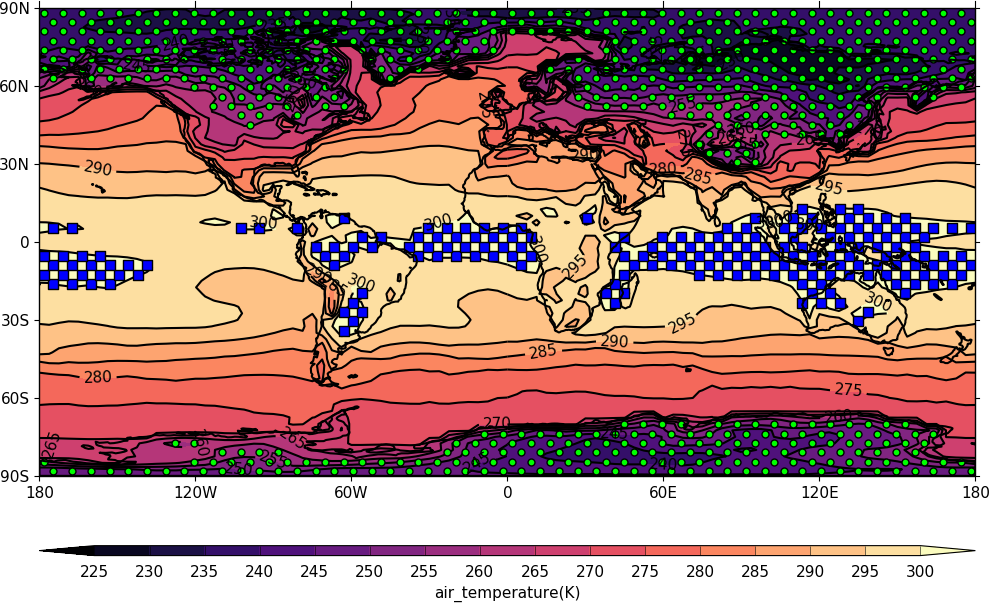Stipple plots¶

Example 17 - stipple plot¶import cf
import cfplot as cfp
g=f.subspace(time=15)
cfp.gopen()
cfp.cscale('magma')
cfp.con(g)
cfp.stipple(f=g, min=220, max=260, size=100, color='#00ff00')
cfp.stipple(f=g, min=300, max=330, size=50, color='#0000ff', marker='s')
cfp.gclose()

Stipple plots are usually used to display significance. The above is a test plot with a temperature field stippled between two different limits. A contour field is displayed underneath to show that the stippling is in the correct regions.

Example 18 - polar stipple plot¶import cf
import cfplot as cfp